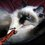# Why metric spaces?

You may have been introduced in your analysis class to the notion of convergence, but only about the convergence of real sequences. If we want to extend this idea for say vectors, complex numbers, functions, tensors, or even sequences of sequences, and to generalize it, we can define for every mathematical object the meaning of convergence. But as you may guess, this isn't very efficient and will quickly get tedious. The other choice is to get more abstract and define a space (which is like a set) that contain all of these objects, and to define convergence there. But since distance is important for convergence, we must be able in this space to determine distances between its points. And ladies and gentlemen, two classes of spaces fit our requirements, the first is the so-called metric spaces, and a more general class of spaces which are called topological spaces.

Another presentation made by T. W. Körner:

If I wish to travel from Cambridge to Edinburgh, then I may be interested in one or more of the following numbers.

1. The distance, in kilometres, from Cambridge to Edinburgh ‘as the crow flies’.
2. The distance, in kilometres, from Cambridge to Edinburgh by road.
3. The time, in minutes, of the shortest journey from Cambridge to Edinburgh by rail.
4. The cost, in pounds, of the cheapest journey from Cambridge to Edinburgh by rail.

Each of these numbers is of interest to someone and none of them is easily obtained from another. However, they do have certain properties in common which we try to isolate in the following definition:

Let $X$ be a metric space with $d:X^2\to\Bbb R$ a function with the following properties:

1. $d(x,y)\geqslant0$ for all $x,y\in X$.
2. $d(x,y)=0$ only and only if $x=y$.
3. $d(x,y)=d(y,x)$ for all $x,y\in X$. (symmetry)
4. $d(x,y)+d(y,z)\geqslant d(x,z)$ for all $x,y,z\in X$. (triangle inequality)Note by حكيم الفيلسوف الضائع
7 years, 2 months ago

This discussion board is a place to discuss our Daily Challenges and the math and science related to those challenges. Explanations are more than just a solution — they should explain the steps and thinking strategies that you used to obtain the solution. Comments should further the discussion of math and science.

When posting on Brilliant:

• Use the emojis to react to an explanation, whether you're congratulating a job well done , or just really confused .
• Ask specific questions about the challenge or the steps in somebody's explanation. Well-posed questions can add a lot to the discussion, but posting "I don't understand!" doesn't help anyone.
• Try to contribute something new to the discussion, whether it is an extension, generalization or other idea related to the challenge.

MarkdownAppears as
*italics* or _italics_ italics
**bold** or __bold__ bold
- bulleted- list
• bulleted
• list
1. numbered2. list
1. numbered
2. list
Note: you must add a full line of space before and after lists for them to show up correctly
paragraph 1paragraph 2

paragraph 1

paragraph 2

[example link](https://brilliant.org)example link
> This is a quote
This is a quote
    # I indented these lines
# 4 spaces, and now they show
# up as a code block.

print "hello world"
# I indented these lines
# 4 spaces, and now they show
# up as a code block.

print "hello world"
MathAppears as
Remember to wrap math in $$ ... $$ or $ ... $ to ensure proper formatting.
2 \times 3 $2 \times 3$
2^{34} $2^{34}$
a_{i-1} $a_{i-1}$
\frac{2}{3} $\frac{2}{3}$
\sqrt{2} $\sqrt{2}$
\sum_{i=1}^3 $\sum_{i=1}^3$
\sin \theta $\sin \theta$
\boxed{123} $\boxed{123}$

Sort by: